## Chi Distribution

The probability density function and cumulative distribution function are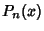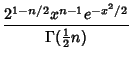(1)(2)

whereis the Regularized Gamma Function.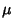(3)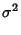(4)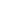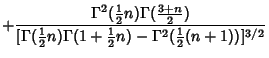(5)(6)

whereis the Mean,the Variance,the Skewness, and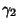the Kurtosis. For, thedistribution is a Half-Normal Distribution with. For, it is a Rayleigh Distribution with.